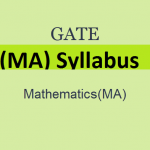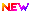# GATE 2020 Mathematics Syllabus (Released) – Get (MA) PDF Here

Latest Applications Open 2020:GATE 2020 Syllabus of Mathematics has been Released. GATE 2020 is a national level exam organized by IIT DELHI. The Engineering Graduation Skill Test (GATE 2020) is organized for admission to PG courses in the field of engineering and technology, specifically ME / M.Tech.

The exam will be held on 1st, 2nd, 8th February and 9th February 2020. See here for complete information about the GATE 2020 Syllabus.

## GATE 2020 Mathematics Syllabus – PDF ReleasedGATE 2020 Mathematics Syllabus has been Released. Click Here to Download Pdf.

## GATE 2020 Mathematics Syllabus

Latest Applications For Various UG & PG Courses Open 2020:

1. Lovely Professional University| Admissions Open for All Courses 2020-21. Apply Now
2. Chandigarh University, Punjab | Admissions Open for All Courses 2020-21. Apply Now
3. Manipal University 2020 Admission Open For UG & PG Courses. Apply Now
4. UPES, Dehradun Enroll Yourself for the Academic Year 2020. Apply Now
5. Pearl Academy No.1 Fashion School – Admissions Open for 2020 batch. Apply Now

Calculus: Finite, countable and uncountable sets, Real number system as a complete ordered field, Archimedean property; Sequences and series, convergence; Limits, continuity, uniform continuity, differentiability, mean value theorems; Riemann integration, Improper integrals;

Functions of two or three variables, continuity, directional derivatives, partial derivatives, total derivative, maxima and minima, saddle point, method of Lagrange’s multipliers; Double and Triple integrals and their applications; Line integrals and Surface integrals, Green’s theorem, Stokes’ theorem, and Gauss divergence theorem.

Linear Algebra: Finite dimensional vector spaces over real or complex fields; Linear transformations and their matrix representations, rank and nullity; systems of linear equations, eigenvalues and eigenvectors, minimal polynomial, Cayley-Hamilton Theorem, diagonalization,

Jordan canonical form, symmetric, skew-symmetric, Hermitian, skew-Hermitian, orthogonal and unitary matrices; Finite dimensional inner product spaces, Gram- Schmidt orthonormalization process, definite forms.

Real Analysis: Metric spaces, connectedness, compactness, completeness; Sequences and series of functions, uniform convergence; Weierstrass approximation theorem; Power series; Functions of several variables: Differentiation, contraction mapping principle,

Inverse and Implicit function theorems; Lebesgue measure, measurable functions; Lebesgue integral, Fatou’s lemma, monotone convergence theorem, dominated convergence theorem.

Complex Analysis: Analytic functions, harmonic functions; Complex integration: Cauchy’s integral theorem and formula; Liouville’s theorem, maximum modulus principle, Morera’s theorem; zeros and singularities; Power series, radius of convergence,

Taylor’s theorem and Laurent’s theorem; residue theorem and applications for evaluating real integrals; Rouche’s theorem, Argument principle, Schwarz lemma; conformal mappings, bilinear transformations.

Ordinary Differential equations: First order ordinary differential equations, existence and uniqueness theorems for initial value problems, linear ordinary differential equations of higher order with constant coefficients; Second-order linear ordinary differential equations with variable coefficients;

Cauchy-Euler equation, method of Laplace transforms for solving ordinary differential equations, series solutions (power series, Frobenius method); Legendre and Bessel functions and their orthogonal properties; Systems of linear first-order ordinary differential equations.

Algebra: Groups, subgroups, normal subgroups, quotient groups, homomorphisms, automorphisms; cyclic groups, permutation groups, Sylow’s theorems, and their applications;

Rings, ideals, prime and maximal ideals, quotient rings, unique factorization domains, Principle ideal domains, Euclidean domains, polynomial rings, and irreducibility criteria; Fields, finite fields, field extensions.

Functional Analysis: Normed linear spaces, Banach spaces, Hahn-Banach theorem, open mapping, and closed graph theorems, the principle of uniform boundedness; Inner-product spaces, Hilbert spaces, orthonormal bases, Riesz representation theorem.

Numerical Analysis: Numerical solutions of algebraic and transcendental equations: bisection, secant method, Newton-Raphson method, fixed-point iteration; Interpolation: error of polynomial interpolation, Lagrange and Newton interpolations; Numerical differentiation;

Numerical integration: Trapezoidal and Simpson’s rules; Numerical solution of a system of linear equations: direct methods (Gauss elimination, LU decomposition), iterative methods (Jacobi and Gauss-Seidel); Numerical solution of initial value problems of ODEs: Euler’s method, Runge-Kutta methods of order 2.

Partial Differential Equations: Linear and quasi-linear first-order partial differential equations, method of characteristics; Second-order linear equations in two variables and their classification; Cauchy, Dirichlet, and Neumann problems;

Solutions of Laplace and wave equations in two-dimensional Cartesian coordinates, interior and exterior Dirichlet problems in polar coordinates; Separation of variables method for solving wave and diffusion equations in one space variable; Fourier series and Fourier transform and Laplace transform methods of solutions for the equations mentioned above.

Topology: Basic concepts of topology, bases, subbases, subspace topology, order topology, product topology, metric topology, connectedness, compactness, countability and separation axioms, Urysohn’s Lemma.

Linear Programming: Linear programming problem and its formulation, convex sets and their properties, graphical method, basic feasible solution, simplex method, two-phase methods; infeasible and unbounded LPP’s, alternate optima; Dual problem and duality theorems; Balanced and unbalanced transportation problems, Vogel’s approximation method for solving transportation problems; Hungarian method for solving assignment problems.

If you any query regarding GATE 2020 Mathematics Syllabus, you can ask your query leave comments below.Lovely Professional University+